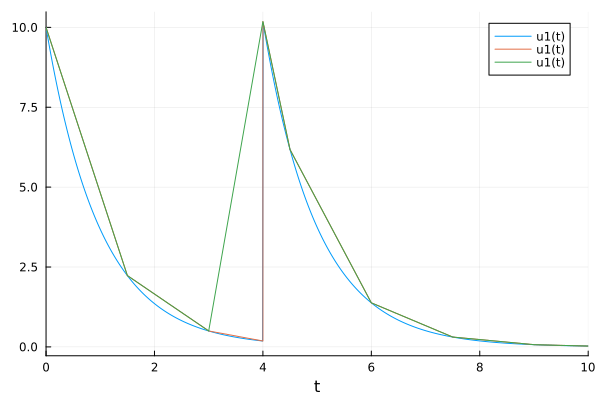# Save_positions parameter in solve's callback function

How does the save_positions parameter in DiscreteCallback work?

In the code example provided in Event Handling and Callback Functions · DifferentialEquations.jl (second example) the following discrete callbacks are written:

using DifferentialEquations
save_positions = (true, true)

cb = DiscreteCallback(condition, affect!, save_positions = save_positions)

save_positions = (false, true)

cb2 = DiscreteCallback(condition2, affect2!, save_positions = save_positions)

cbs = CallbackSet(cb, cb2)


What does the tuple boolean values specify? Why in this example is one set to (true, true) and the other to (false, true)?

thanks!

The tuple specifies whether to save a result (i.e. the value of the state variables (u)) at the timestep before (first boolean) and after the event (second boolean).

That’s important when you have discontinuities present, let’s say for example when your affect function changes u at event time.

at a discontinuity that, for instance, adds a dose at a certain time – wouldn’t I want this to be (false, true)? The code example shows that it should be (true, true).

It depends. If you do (false, true) you will only save the value after the discontinuity. Let’s look at a few plots.

using DifferentialEquations, Plots
function f(du, u, p, t)
du = -u
end
u0 = [10.0]
const V = 1
prob = ODEProblem(f, u0, (0.0, 10.0))
sol = solve(prob, Tsit5())

condition(u, t, integrator) = t == 4
affect!(integrator) = integrator.u += 10
cb = DiscreteCallback(condition, affect!)
cb2 = DiscreteCallback(condition, affect!, save_positions = (false, true))

sol = solve(prob, Tsit5(), callback = cb, tstops = [4.0])
plot(sol)

sol = solve(prob, Tsit5(), callback = cb, saveat = 1.5, tstops = [4.0])
plot!(sol)

sol = solve(prob, Tsit5(), callback = cb2, saveat = 1.5, tstops = [4.0])
plot!(sol)

savefig("plot.png")In the first case in blue, we have the best solution. Then we have a solution that isn’t saved very often, but does save before and after the callback. Then we only save after the callback. The discontinuity is not well resolved.

For a DiscreteCallback where you know the points ahead of time this isn’t a big deal. This is more of a matter for ContinuousCallbacks causing discontinuities at unknown times, like in the bouncing ball example.

2 Likes